## A strange zero divisor

Let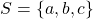be a three element set. Equip its power set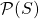with two operations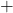anddefined as:and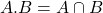, for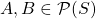. Then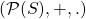is a ring. The zero-divisors in this ring are the singleton subsets and the two element subsets.

Recall that an element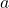in a ring is a zero-divisor if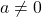and there exists another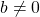for which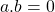(technically, a left zero-divisor).

The zero element inis obviously the empty set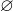. In addition, any singleton subset has an empty intersection with another singleton subset: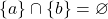(we’ve assumed implicitly that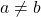).

Consider a two element subset, e.g.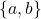. We have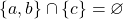. Similarly,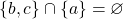and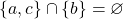. So the two element subsets are also zero-divisors.

The entire set itself, namely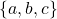is not a zero-divisor.

What is strange about these zero divisors as the title suggests? Nothing.

This concludes today’s snippet. Thanks for visiting!